登  录风  格绿草青青青青苹果蓝色经典佳人有约蓝色梦想蓝天白云蓝色狂想蓝色魅力魅力星空
 »  游客当前位置：  论坛首页 »  自由讨论区 »  AI.人工智能讨论区 »总帖数 1 每页帖数 10 1/1页 1
0查看: 1125 | 回复: 0 主题： [转帖]从决策树到随机森林：树型算法的原理与实现huang.wang 高级会员等级：中将 经验：17623 发帖：407 精华：1 注册：1970-1-1 状态：离线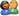加好友发消息

#### 决策树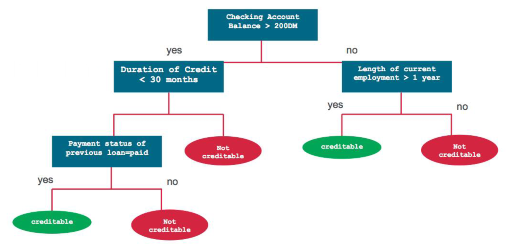CART 模型

CART 模型包括选择输入变量和那些变量上的分割点，直到创建出适当的树。使用贪婪算法（greedy algorithm）选择使用哪个输入变量和分割点，以使成本函数（cost function）最小化。

ID3：Iterative Dichotomiser 3

C4.5：ID3 算法的改进

CHAID：Chi-squared Automatic Interaction Detector

MARS：决策树的扩展式，以更好地解决数值型预测。

1. 把预测器空间，即一系列可能值 X_1，X_2，...，X_p 分成 J 个不同的且非重叠的区域 R_1，R_2，...，R_J。

2. 对进入区域 R_J 的每一个样本观测值都进行相同的预测，该预测就是 R_J 中训练样本预测值的均值。Gini 指数可以衡量 k 个类别的总方差，它一般定义为：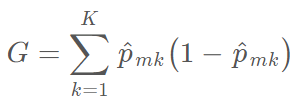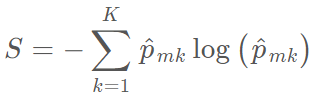import pandas as pdimport numpy as npfrom plotnine import *import matplotlib.pyplot as pltfrom sklearn.preprocessing import LabelEncoderfrom sklearn_pandas import DataFrameMapperfrom sklearn.tree import DecisionTreeClassifierfrom sklearn.ensemble import RandomForestClassifier

df_train_set.drop('fnlgwt', axis=1, inplace=True)

df_test_set.drop('fnlgwt', axis=1, inplace=True)#replace the special character to "Unknown"for i in df_train_set.columns:

df_train_set[i].replace(' ?', 'Unknown', inplace=True)

df_test_set[i].replace(' ?', 'Unknown', inplace=True)for col in df_train_set.columns:if df_train_set[col].dtype != 'int64':

df_train_set[col] = df_train_set[col].apply(lambda val: val.replace(" ", ""))

df_train_set[col] = df_train_set[col].apply(lambda val: val.replace(".", ""))

df_test_set[col] = df_test_set[col].apply(lambda val: val.replace(" ", ""))

df_test_set[col] = df_test_set[col].apply(lambda val: val.replace(".", ""))

df_train_set.drop(["Country", "Education"], axis=1, inplace=True)

df_test_set.drop(["Country", "Education"], axis=1, inplace=True)

Age 和 EdNum 列是数值型的，我们可以将连续数值型转化为更高效的方式，例如将年龄换为 10 年的整数倍，教育年限换为 5 年的整数倍，实现的代码如下：

colnames = list(df_train_set.columns)

colnames.remove('Age')

colnames.remove('EdNum')

colnames = ['AgeGroup', 'Education'] + colnames

labels = ["{0}-{1}".format(i, i + 9) for i in range(0, 100, 10)]

df_train_set['AgeGroup'] = pd.cut(df_train_set.Age, range(0, 101, 10), right=False, labels=labels)

df_test_set['AgeGroup'] = pd.cut(df_test_set.Age, range(0, 101, 10), right=False, labels=labels)

labels = ["{0}-{1}".format(i, i + 4) for i in range(0, 20, 5)]

df_train_set['Education'] = pd.cut(df_train_set.EdNum, range(0, 21, 5), right=False, labels=labels)

df_test_set['Education'] = pd.cut(df_test_set.EdNum, range(0, 21, 5), right=False, labels=labels)

df_train_set = df_train_set[colnames]

df_test_set = df_test_set[colnames]

df_train_set.Income.value_counts()

<=50K    24720

>50K      7841

Name: Income, dtype: int64

df_test_set.Income.value_counts()

<=50K    12435

>50K      3846

Name: Income, dtype: int64

### EDA

（Relationships）和婚姻状况（MaritalStatus）特征是如何相互关联的。

df_train_set.Income.value_counts()

<=50K    24720

>50K      7841

Name: Income, dtype: int64

df_test_set.Income.value_counts()

<=50K    12435

>50K      3846

Name: Income, dtype: int64(ggplot(df_train_set, aes(x = "Education", fill = "Income"))+ geom_bar(position="fill")+ theme(axis_text_x = element_text(angle = 60, hjust = 1))+ facet_wrap('~AgeGroup'))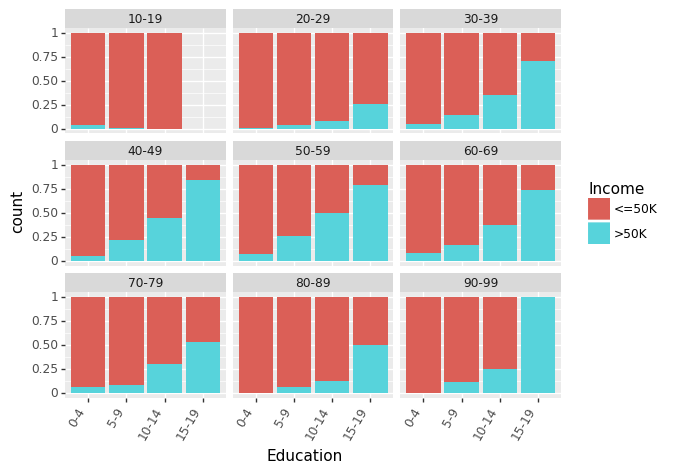(ggplot(df_train_set, aes(x = "Education", fill = "Income"))+ geom_bar(position="fill")+ theme(axis_text_x = element_text(angle = 60, hjust = 1))+ facet_wrap('~AgeGroup'))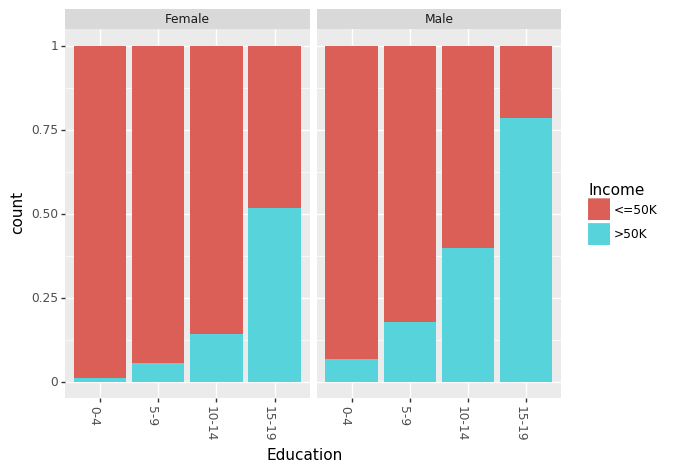(ggplot(df_train_set, aes(x = "Education", fill = "Income"))+ geom_bar(position="fill")+ theme(axis_text_x = element_text(angle = 60, hjust = 1))+ facet_wrap('~AgeGroup'))(ggplot(df_train_set, aes(x="Income", y="CapitalGain"))+ geom_jitter(position=position_jitter(0.1)))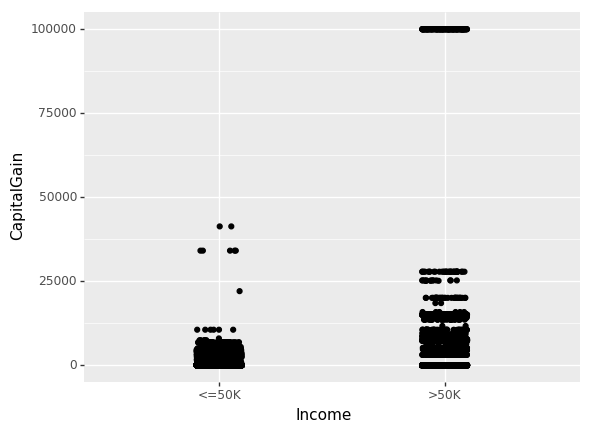(ggplot(df_train_set, aes(x="Income", y="CapitalLoss"))+ geom_jitter(position=position_jitter(0.1)))### 树分类器

mapper = DataFrameMapper([('AgeGroup', LabelEncoder()),('Education', LabelEncoder()),('Workclass', LabelEncoder()),('MaritalStatus', LabelEncoder()),('Occupation', LabelEncoder()),('Relationship', LabelEncoder()),('Race', LabelEncoder()),('Sex', LabelEncoder()),('Income', LabelEncoder())], df_out=True, default=None)

cols = list(df_train_set.columns)

cols.remove("Income")

cols = cols[:-3] + ["Income"] + cols[-3:]

df_train = mapper.fit_transform(df_train_set.copy())

df_train.columns = cols

df_test = mapper.transform(df_test_set.copy())

df_test.columns = cols

cols.remove("Income")

x_train, y_train = df_train[cols].values, df_train["Income"].values

x_test, y_test = df_test[cols].values, df_test["Income"].values

treeClassifier = DecisionTreeClassifier()

treeClassifier.fit(x_train, y_train)

treeClassifier.score(x_test, y_test)

import itertoolsfrom sklearn.metrics import confusion_matrixdef plot_confusion_matrix(cm, classes, normalize=False):"""

This function prints and plots the confusion matrix.

Normalization can be applied by setting `normalize=True`.

"""

cmap = plt.cm.Blues

title = "Confusion Matrix"if normalize:

cm = cm.astype('float') / cm.sum(axis=1)[:, np.newaxis]

cm = np.around(cm, decimals=3)

plt.imshow(cm, interpolation='nearest', cmap=cmap)

plt.title(title)

plt.colorbar()

tick_marks = np.arange(len(classes))

plt.xticks(tick_marks, classes, rotation=45)

plt.yticks(tick_marks, classes)

thresh = cm.max() / 2.for i, j in itertools.product(range(cm.shape), range(cm.shape)):

plt.text(j, i, cm[i, j],

horizontalalignment="center",

color="white" if cm[i, j] > thresh else "black")

plt.tight_layout()

plt.ylabel('True label')

plt.xlabel('Predicted label')

y_pred = treeClassifier.predict(x_test)

cfm = confusion_matrix(y_test, y_pred, labels=[0, 1])

plt.figure(figsize=(10,6))

plot_confusion_matrix(cfm, classes=["<=50K", ">50K"], normalize=True)from sklearn.model_selection import GridSearchCV

parameters = {'max_features':(None, 9, 6),'max_depth':(None, 24, 16),'min_samples_split': (2, 4, 8),'min_samples_leaf': (16, 4, 12)}

clf = GridSearchCV(treeClassifier, parameters, cv=5, n_jobs=4)

clf.fit(x_train, y_train)

clf.best_score_, clf.score(x_test, y_test), clf.best_params_

(0.85934092933263717,

0.85897672133161351,

{'max_depth': 16,

'max_features': 9,

'min_samples_leaf': 16,

'min_samples_split': 8})

y_pred = clf.predict(x_test)

cfm = confusion_matrix(y_test, y_pred, labels=[0, 1])

plt.figure(figsize=(10,6))

plot_confusion_matrix(cfm, classes=["<=50K", ">50K"], normalize=True)### 袋装（Bootstrap Aggregating——Bagging）Out-of-Bag（OOB）误差

Bagging 方法最大的优势是我们可以不通过交叉验证而求得测试误差。回想一下，Bagging 方法的精髓是多棵树可以重复地拟合观察样本的自助子集。平均而言，每一个袋装树可以利用 2/3 的观察样本。而剩下的 1/3 观察样本就可以称为 out-of-bag (OOB) 观察样本，它们并不会拟合一一棵给定袋装树。我们可以使用每一棵树的 OOB 观察样本而计算第 i 个观察样本的预测值，这将会导致大约有 B/3 的预测值可以预测第 i 个观察样本。现在我们可以使用和 Bagging（平均回归和大多数投票分类）类似的聚集技术，我们能获得第 i 个观察样本的单一预测值。我们可以用这种方式获得 n 个观察样本的 OOB 预测，因此总体的 OOB MSE（回归问题）和分类误差率（分类问题）就能计算出来。OOB 误差结果是 Bagging 模型测试误差的有效估计，因为每一个样本的预测值都是仅仅使用不会进行拟合训练模型的样本。

### 随机森林模型

rclf = RandomForestClassifier(n_estimators=500)

rclf.fit(x_train, y_train)

rclf.score(x_test, y_test)

rclf = RandomForestClassifier(n_estimators=500)

rclf.fit(x_train, y_train)

rclf.score(x_test, y_test)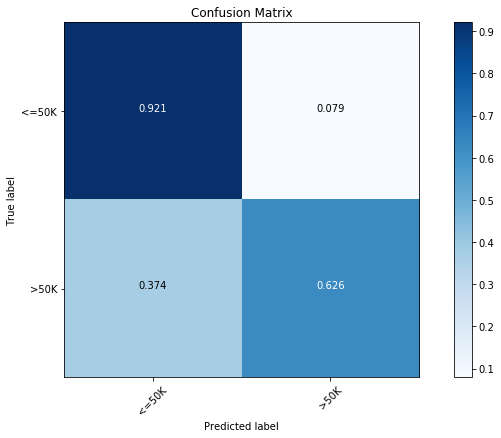importances = rclf.feature_importances_

indices = np.argsort(importances)

cols = [cols[x] for x in indices]

plt.figure(figsize=(10,6))

plt.title('Feature Importances')

plt.barh(range(len(indices)), importances[indices], color='b', align='center')

plt.yticks(range(len(indices)), cols)

plt.xlabel('Relative Importance')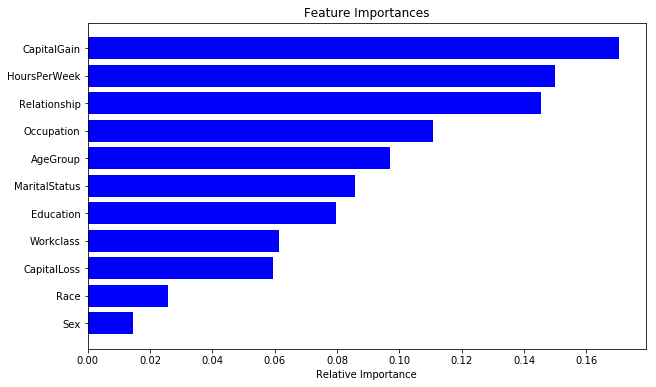parameters = {'n_estimators':(100, 500, 1000),'max_depth':(None, 24, 16),'min_samples_split': (2, 4, 8),'min_samples_leaf': (16, 4, 12)}

clf = GridSearchCV(RandomForestClassifier(), parameters, cv=5, n_jobs=8)

clf.fit(x_train, y_train)

clf.best_score_, clf.best_params_

0.86606676699118579

{'max_depth': 24, 'min_samples_leaf': 4, 'min_samples_split': 4, 'n_estimators': 1000}

0.86606676699118579

{'max_depth': 24,

'min_samples_leaf': 4,

'min_samples_split': 4,

'n_estimators': 1000}

rclf2 = RandomForestClassifier(n_estimators=1000,max_depth=24,min_samples_leaf=4,min_samples_split=8)

rclf2.fit(x_train, y_train)

y_pred = rclf2.predict(x_test)

cfm = confusion_matrix(y_test, y_pred, labels=[0, 1])

plt.figure(figsize=(10,6))

plot_confusion_matrix(cfm, classes=["<=50K", ">50K"], normalize=True)importances = rclf2.feature_importances_
indices = np.argsort(importances)
cols = [cols[x] for x in indices]
plt.figure(figsize=(10,6))
plt.title('Feature Importances')
plt.barh(range(len(indices)), importances[indices], color='b', align='center')
plt.yticks(range(len(indices)), cols)
plt.xlabel('Relative Importance')本版精华 热门帖子

——来自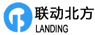操作    引用/回复总帖数 1 每页帖数 10 1/1页 1请输入验证码：您需要登录后才可以回帖 登录 | 注册技术讨论